# XBRL FORMULA IN PRACTICE IN REGULATORY ENVIRONMENTS EXPERIENCE

• Slides: 89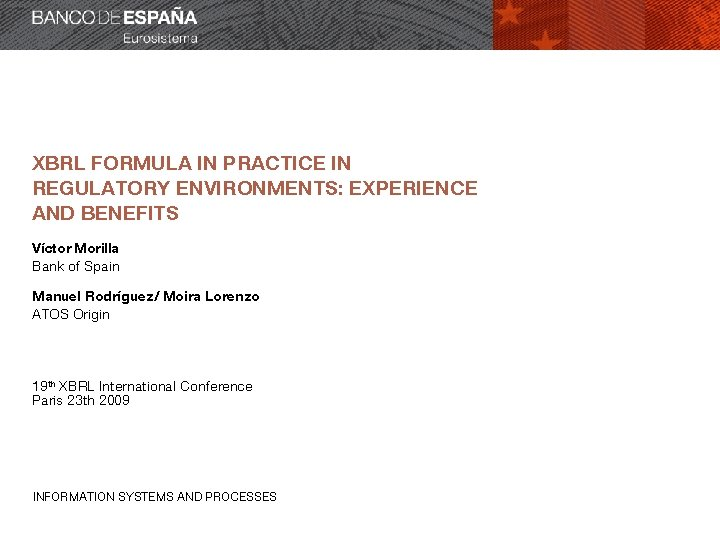XBRL FORMULA IN PRACTICE IN REGULATORY ENVIRONMENTS: EXPERIENCE AND BENEFITS Víctor Morilla Bank of Spain Manuel Rodríguez/ Moira Lorenzo ATOS Origin 19 th XBRL International Conference Paris 23 th 2009 INFORMATION SYSTEMS AND PROCESSES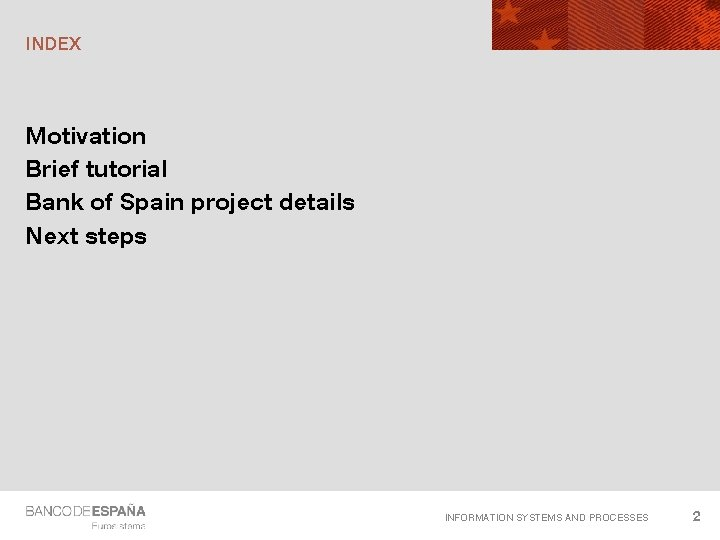INDEX Motivation Brief tutorial Bank of Spain project details Next steps INFORMATION SYSTEMS AND PROCESSES 2MOTIVATION INFORMATION SYSTEMS AND PROCESSES 3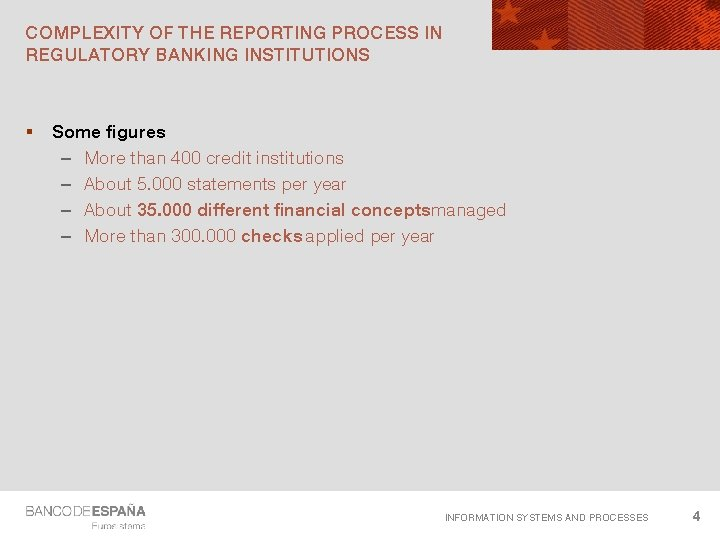COMPLEXITY OF THE REPORTING PROCESS IN REGULATORY BANKING INSTITUTIONS § Some figures – More than 400 credit institutions – About 5. 000 statements per year – About 35. 000 different financial conceptsmanaged – More than 300. 000 checks applied per year INFORMATION SYSTEMS AND PROCESSES 4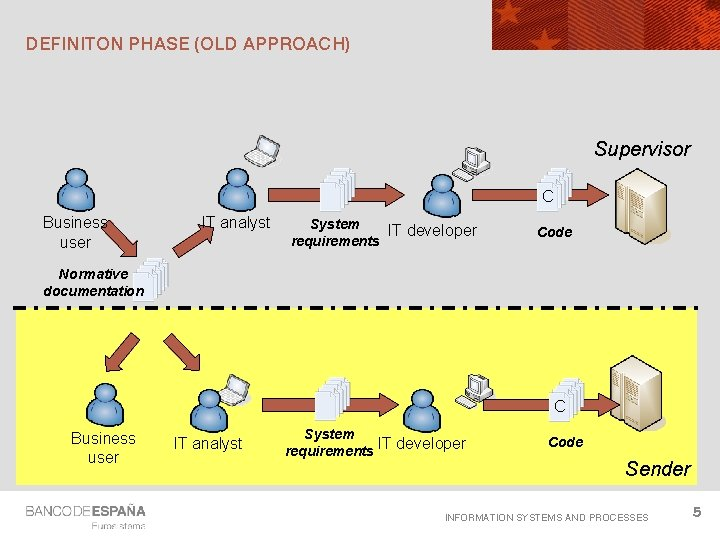DEFINITON PHASE (OLD APPROACH) Supervisor C Business user IT analyst System IT developer requirements Code Normative documentation C Business user IT analyst System requirements IT developer Code Sender INFORMATION SYSTEMS AND PROCESSES 5FILING PROCESS TIMELINE (OLD APPROACH) Supervisor Analysis Notification Errors detected Validation OK Data extraction & transformation Analysis and corrections Sender INFORMATION SYSTEMS AND PROCESSES 6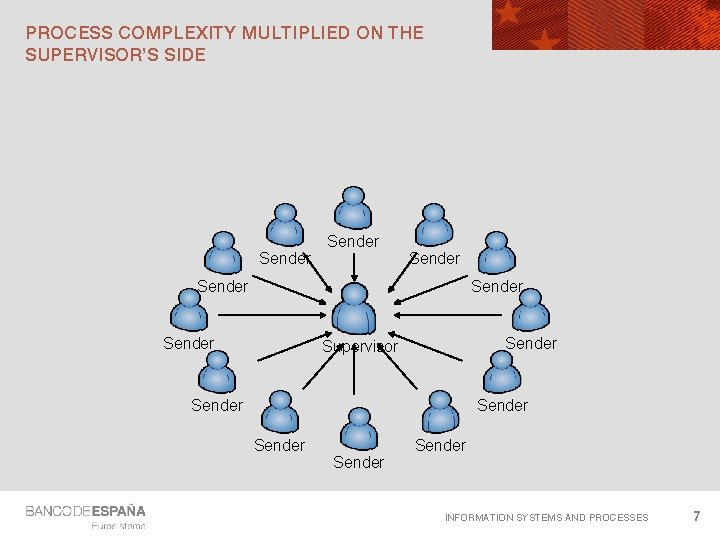PROCESS COMPLEXITY MULTIPLIED ON THE SUPERVISOR’S SIDE Sender Sender Supervisor Sender Sender INFORMATION SYSTEMS AND PROCESSES 7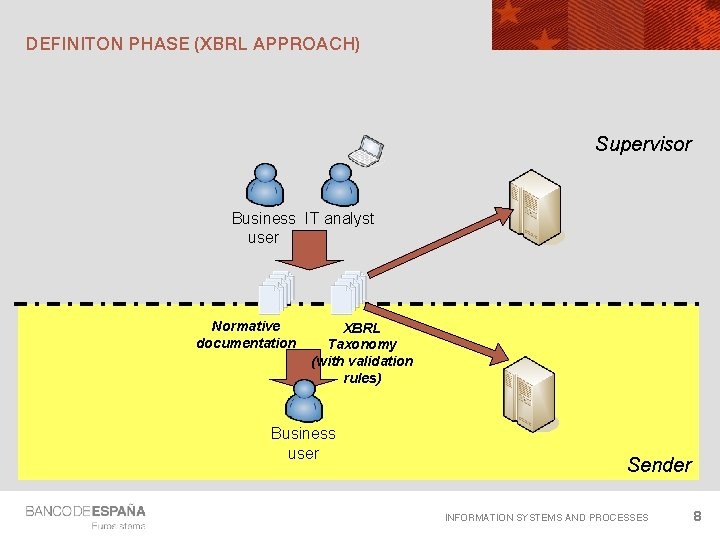DEFINITON PHASE (XBRL APPROACH) Supervisor Business IT analyst user Normative documentation XBRL Taxonomy (with validation rules) Business user Sender INFORMATION SYSTEMS AND PROCESSES 8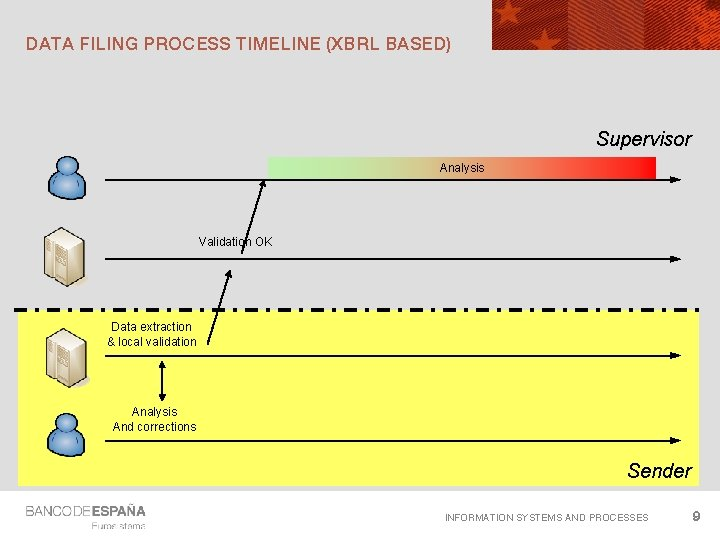DATA FILING PROCESS TIMELINE (XBRL BASED) Supervisor Analysis Validation OK Data extraction & local validation Analysis And corrections Sender INFORMATION SYSTEMS AND PROCESSES 9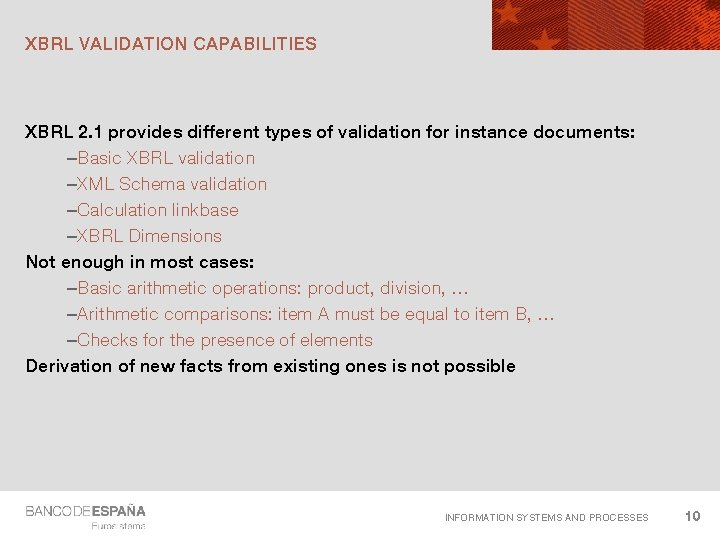XBRL VALIDATION CAPABILITIES XBRL 2. 1 provides different types of validation for instance documents: –Basic XBRL validation –XML Schema validation –Calculation linkbase –XBRL Dimensions Not enough in most cases: –Basic arithmetic operations: product, division, … –Arithmetic comparisons: item A must be equal to item B, … –Checks for the presence of elements Derivation of new facts from existing ones is not possible INFORMATION SYSTEMS AND PROCESSES 10WHY A NEW LANGUAGE? Why not using existing languages like. . . -JAVA, C#, C++ or Cobol -XML based languages like XSLT, XQuery or Schematron Key requirements –Intuitive –Maintainable –Documentation capabilities –Extensible INFORMATION SYSTEMS AND PROCESSES 11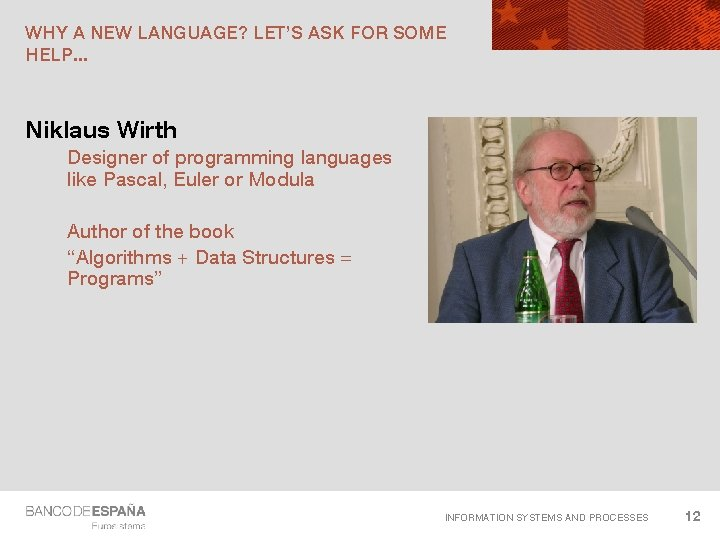WHY A NEW LANGUAGE? LET’S ASK FOR SOME HELP. . . Niklaus Wirth Designer of programming languages like Pascal, Euler or Modula Author of the book “Algorithms + Data Structures = Programs” INFORMATION SYSTEMS AND PROCESSES 12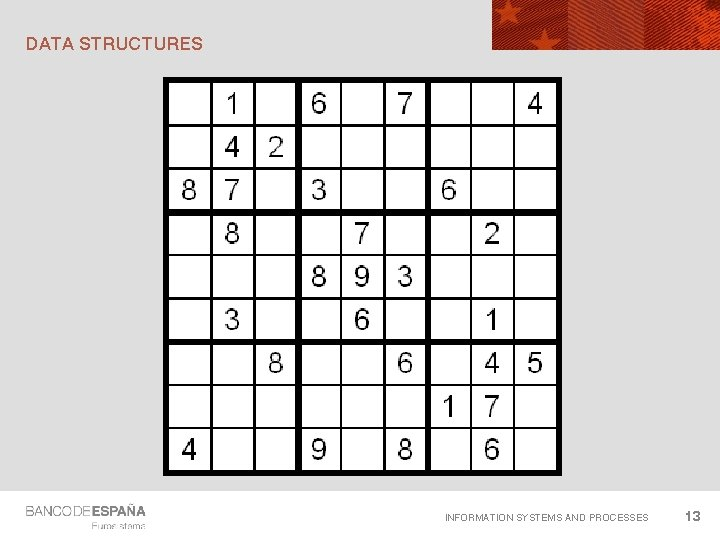DATA STRUCTURES INFORMATION SYSTEMS AND PROCESSES 13DATA STRUCTURES 1 2 3 4 5 6 7 8 9 10 11 12 78 79 80 81 . . . evn : checks that a set of cells has at least one 1, 2, . . . , 9 evn(1, 2, . . . , 9) and evn(10, 11, . . . , 18) and evn(19, 20, . . . , 27) and. . . evn(73, 74, . . . , 81) and evn(1, 10, . . . , 73) and evn(2, 11, . . . , 74) and. . . evn(9, 27, . . . , 81) and evn(1, 2, 3, 10, 11, 12, 19, 20, 21) and. . . for i in (0. . . 8) evn(1 + i*9, 2 + i*9, . . . , 9 + i*9) for i in (0. . 8) evn(1 + i, 10 + i, . . . , 73 + i) for i in (0. . 2) for j in (0. . 2) evn(1 + i + j*9, 2 + i + j*9, . . . ) INFORMATION SYSTEMS AND PROCESSES 14DATA STRUCTURES Column group 1 1 2 3 Column group 2 4 5 6 Column group 3 7 8 9 Row 1 Row group 1 Row 2 Row 3 Row 4 Row group 2 Row 5 Row 6 Row 7 Row group 3 Row 8 Row 9 INFORMATION SYSTEMS AND PROCESSES 15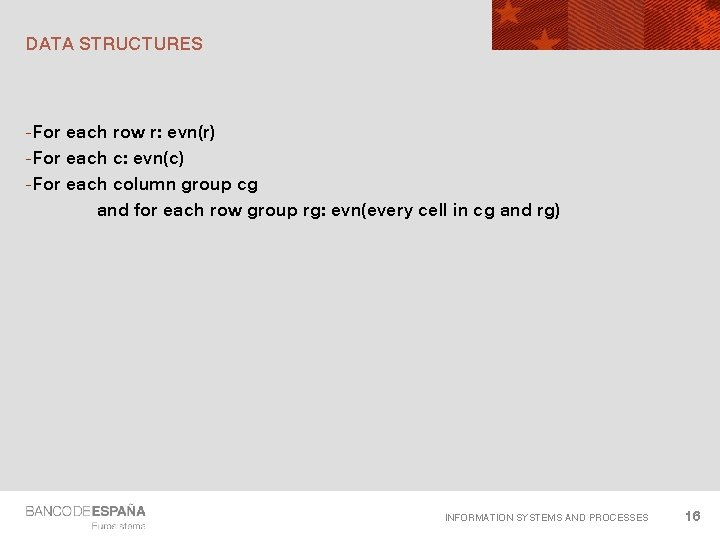DATA STRUCTURES -For each row r: evn(r) -For each c: evn(c) -For each column group cg and for each row group rg: evn(every cell in cg and rg) INFORMATION SYSTEMS AND PROCESSES 16DATA STRUCTURE OF XSLT, XQUERY OR SCHEMATRON INFORMATION SYSTEMS AND PROCESSES 17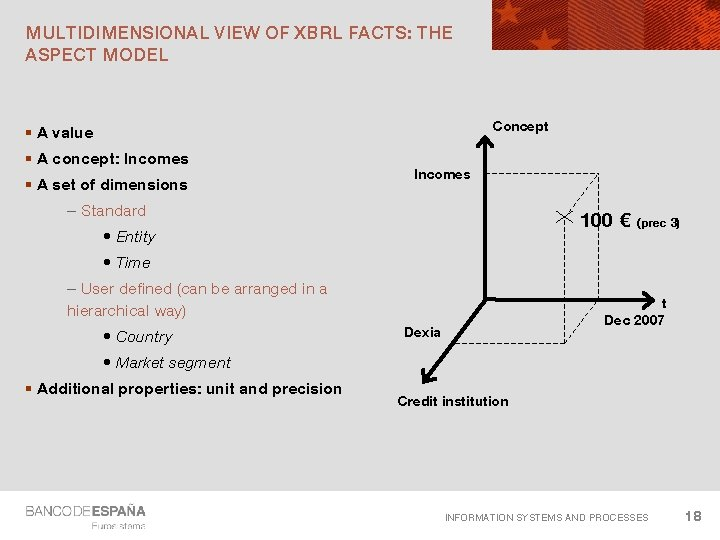MULTIDIMENSIONAL VIEW OF XBRL FACTS: THE ASPECT MODEL Concept § A value § A concept: Incomes § A set of dimensions Incomes – Standard 100 € • Entity (prec 3) • Time – User defined (can be arranged in a hierarchical way) • Country t Dec 2007 Dexia • Market segment § Additional properties: unit and precision Credit institution INFORMATION SYSTEMS AND PROCESSES 18Formula Author XBRL Formula Processor INFORMATION SYSTEMS AND PROCESSES 19BRIEF TUTORIAL (LEARN XBRL FORMULA IN 50 MINUTES) INFORMATION SYSTEMS AND PROCESSES 20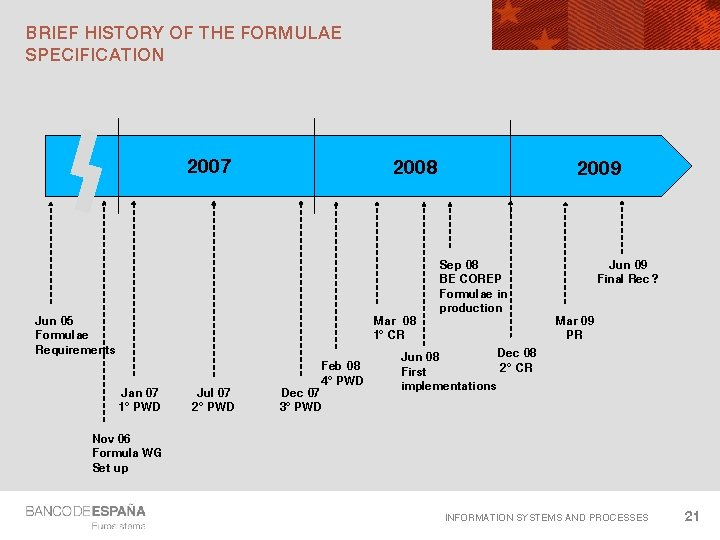BRIEF HISTORY OF THE FORMULAE SPECIFICATION 2007 2008 Jun 05 Formulae Requirements Mar 08 1º CR Jan 07 1º PWD Jul 07 2º PWD Feb 08 4º PWD Dec 07 3º PWD 2009 Sep 08 BE COREP Formulae in production Jun 09 Final Rec ? Mar 09 PR Dec 08 Jun 08 2º CR First implementations Nov 06 Formula WG Set up INFORMATION SYSTEMS AND PROCESSES 21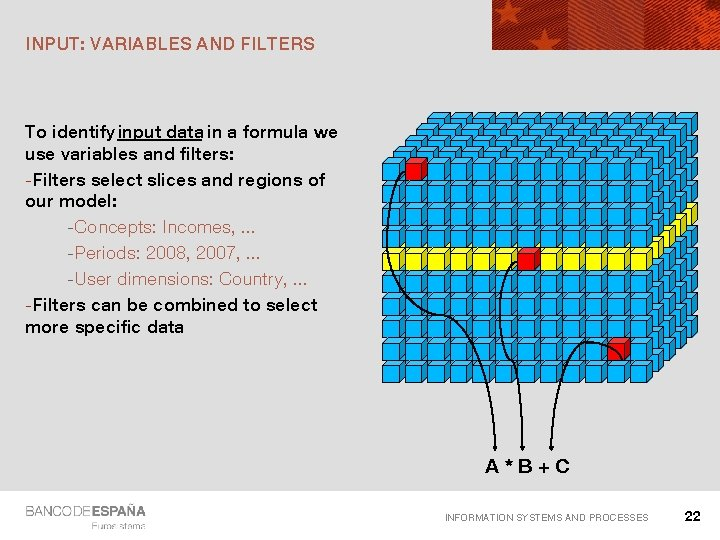INPUT: VARIABLES AND FILTERS To identify input data in a formula we use variables and filters: -Filters select slices and regions of our model: -Concepts: Incomes, . . . -Periods: 2008, 2007, . . . -User dimensions: Country, . . . -Filters can be combined to select more specific data A*B+C INFORMATION SYSTEMS AND PROCESSES 22OUTPUT: XPATH EXPRESSIONS (\$a + \$b) * 2. 5 abs(\$x + \$y) = \$z XPath 2. 0: W 3 C Recommendation (since January 2007) Expression language used by XSLT, XQuery and Schematron We can combine: Arithmetic expressions Logical expressions Conditional expressions … Defines a set of standard operators and functions: +, -, *, div, mod, =, !=, <, >, and, or, … abs, ceiling, floor, concat, upper-case, … INFORMATION SYSTEMS AND PROCESSES 23TYPES OF FORMULAE Formulae to produce new facts from existing ones Assertions to verify a condition: true or false Value assertions Existence assertions Consistency assertions INFORMATION SYSTEMS AND PROCESSES 24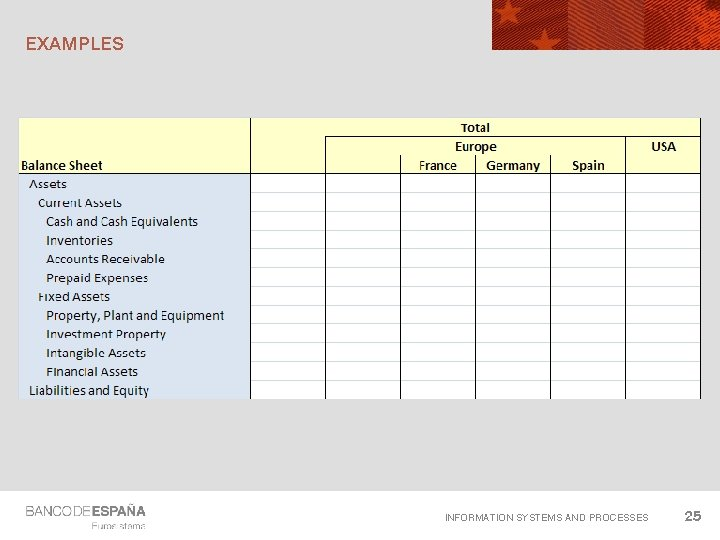EXAMPLES INFORMATION SYSTEMS AND PROCESSES 25EXAMPLE 1 “Assets“ equal to “Liabilities and Equity” Two input variables: \$assets: Concept name: t: Assets \$liab. Eq: Concept name: p-cm-mr: Net. Positions. Short Test : “\$assets = \$liab. Eq” INFORMATION SYSTEMS AND PROCESSES 26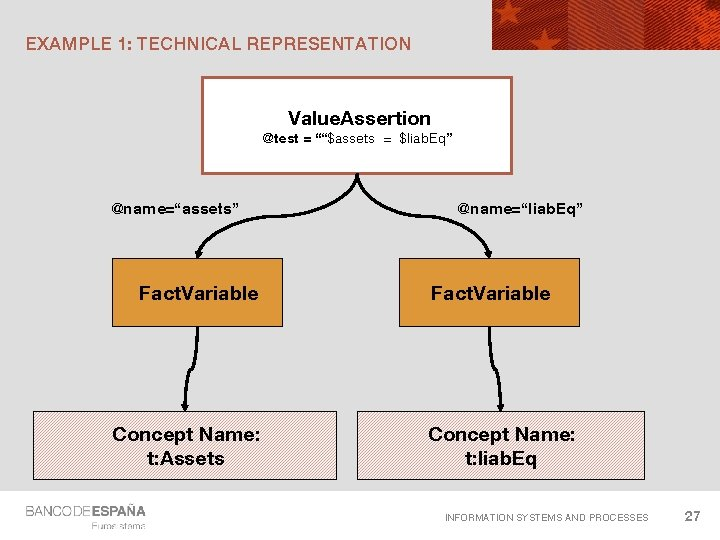EXAMPLE 1: TECHNICAL REPRESENTATION Value. Assertion @test = ““\$assets = \$liab. Eq” @name=“assets” Fact. Variable Concept Name: t: Assets @name=“liab. Eq” Fact. Variable Concept Name: t: liab. Eq INFORMATION SYSTEMS AND PROCESSES 27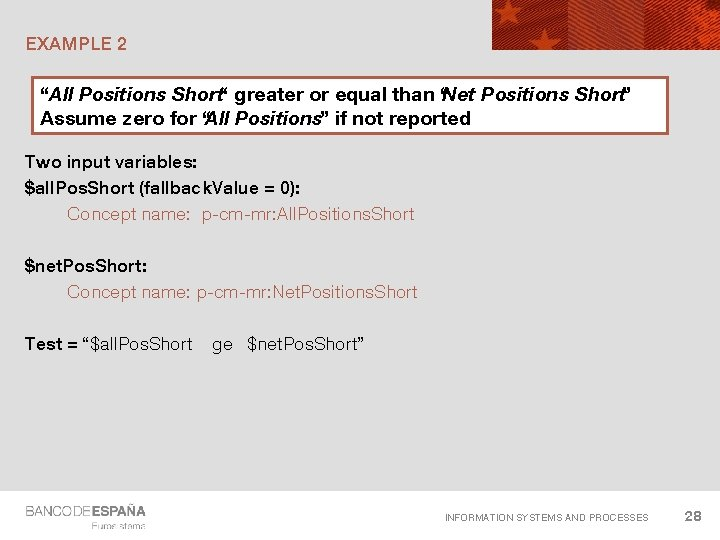EXAMPLE 2 “All Positions Short“ greater or equal than “Net Positions Short” Assume zero for “All Positions” if not reported Two input variables: \$all. Pos. Short (fallback. Value = 0): Concept name: p-cm-mr: All. Positions. Short \$net. Pos. Short: Concept name: p-cm-mr: Net. Positions. Short Test = “\$all. Pos. Short ge \$net. Pos. Short” INFORMATION SYSTEMS AND PROCESSES 28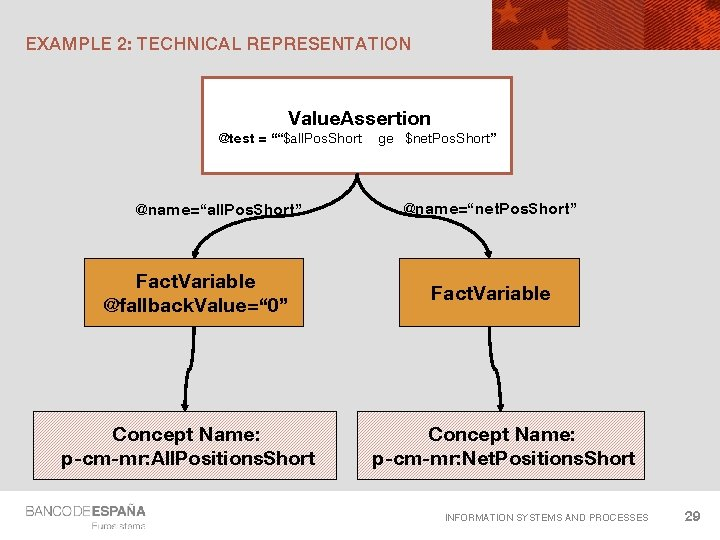EXAMPLE 2: TECHNICAL REPRESENTATION Value. Assertion @test = ““\$all. Pos. Short @name=“all. Pos. Short” Fact. Variable @fallback. Value=“ 0” Concept Name: p-cm-mr: All. Positions. Short ge \$net. Pos. Short” @name=“net. Pos. Short” Fact. Variable Concept Name: p-cm-mr: Net. Positions. Short INFORMATION SYSTEMS AND PROCESSES 29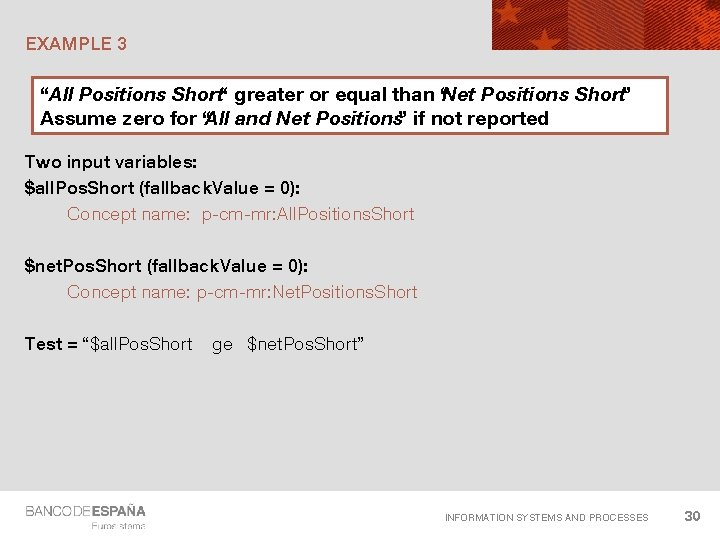EXAMPLE 3 “All Positions Short“ greater or equal than “Net Positions Short” Assume zero for “All and Net Positions” if not reported Two input variables: \$all. Pos. Short (fallback. Value = 0): Concept name: p-cm-mr: All. Positions. Short \$net. Pos. Short (fallback. Value = 0): Concept name: p-cm-mr: Net. Positions. Short Test = “\$all. Pos. Short ge \$net. Pos. Short” INFORMATION SYSTEMS AND PROCESSES 30EXAMPLE 4 A “All Positions Short“ greater or equal than “Net Positions Short” For Total Equities in Trading Book Two input variables: \$all. Pos. Short : Concept name: p-cm-mr: All. Positions. Short Explicit dimension: d-mr: Market. Risk. Dimension= d-mr: MRisk. SAEQUTotal \$net. Pos. Short : Concept name: p-cm-mr: Net. Positions. Short Explicit dimension: d-mr: Market. Risk. Dimension= d-mr: MRisk. SAEQUTotal Test = “\$all. Pos. Short ge \$net. Pos. Short” INFORMATION SYSTEMS AND PROCESSES 31COMBINING FILTERS Two or more filters produce the “intersection” of desired values (they are combined using an logical “AND”) Boolean filters can be used for more complex combinations: - OR filter - AND filter The attribute “complement” in arcs to filters can be used to obtain the negation of the filter linked (this attribute has been assumed to be “false” in examples). INFORMATION SYSTEMS AND PROCESSES 32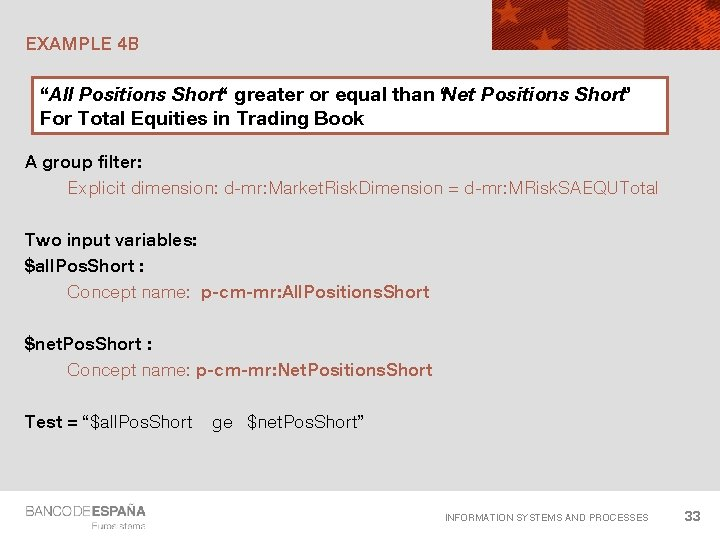EXAMPLE 4 B “All Positions Short“ greater or equal than “Net Positions Short” For Total Equities in Trading Book A group filter: Explicit dimension: d-mr: Market. Risk. Dimension = d-mr: MRisk. SAEQUTotal Two input variables: \$all. Pos. Short : Concept name: p-cm-mr: All. Positions. Short \$net. Pos. Short : Concept name: p-cm-mr: Net. Positions. Short Test = “\$all. Pos. Short ge \$net. Pos. Short” INFORMATION SYSTEMS AND PROCESSES 33EXAMPLE 5 A “All Positions Short“ greater or equal than “Net Positions Short” For General risk and for Specific risk A group filter: Explicit dimension: d-mr: Market. Risk. Dimension d-mr: MRisk. SAEQUGeneral. Risk d-mr: MRisk. SAEQUSpecific. Risk Two input variables: \$all. Pos. Short : Concept name: p-cm-mr: All. Positions. Short \$net. Pos. Short : Concept name: p-cm-mr: Net. Positions. Short Test = “\$all. Pos. Short ge \$net. Pos. Short” INFORMATION SYSTEMS AND PROCESSES 34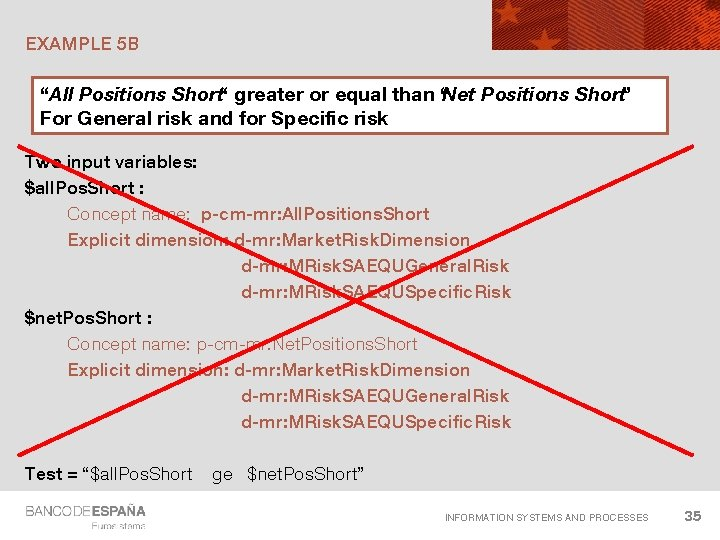EXAMPLE 5 B “All Positions Short“ greater or equal than “Net Positions Short” For General risk and for Specific risk Two input variables: \$all. Pos. Short : Concept name: p-cm-mr: All. Positions. Short Explicit dimension: d-mr: Market. Risk. Dimension d-mr: MRisk. SAEQUGeneral. Risk d-mr: MRisk. SAEQUSpecific. Risk \$net. Pos. Short : Concept name: p-cm-mr: Net. Positions. Short Explicit dimension: d-mr: Market. Risk. Dimension d-mr: MRisk. SAEQUGeneral. Risk d-mr: MRisk. SAEQUSpecific. Risk Test = “\$all. Pos. Short ge \$net. Pos. Short” INFORMATION SYSTEMS AND PROCESSES 35EXAMPLE 6 Any “Net Positions Short“ less than “Cap Requirements for equities in trading books” Two input variables: \$cap. Req : Concept name: p-cm-mr: Market. Risk. Capital. Requirements Explicit dimension: d-mr: Market. Risk. Dimension= d-mr: MRisk. SAEQUTotal \$net. Pos. Short : Concept name: p-cm-mr: Net. Positions. Short Test = “\$net. Pos. Short lt \$cap. Req ” INFORMATION SYSTEMS AND PROCESSES 36THE IMPLICIT FILTER Three steps in the evaluation of a set of variables: Each variable is evaluated individually to obtain the list of all candidate values \$a = (a 1, a 2, a 3) \$b = (b 1, b 2) \$c = (c 1, c 2) The product obtained: a 1 Cartesian a 1 a 1 is a 2 a 2 a 3 a 3 b 1 b 1 b 2 b 2 b 1 b 2 c 1 c 2 c 1 c 2 The implicit filter discards every combination that doesn’t verifies the following condition: INFORMATION SYSTEMS AND PROCESSES 37THE IMPLICIT FILTER For every pair of variables of a formula / assertion, the value of each aspect that is not explicitly filtered* by a variable filter must be the same So, combinations that mix different values for aspects not explicitly filtered are not allowed More precisely: aspects not covered. An aspect is covered for a variable if that variables has a variable filter for that aspect with the attribute @cover with a value of true. In this tutorial, it is assumed that every variable filter has this attribute set to true. Group filters don’t cover aspects. So, group filters are ignored by this rule INFORMATION SYSTEMS AND PROCESSES 38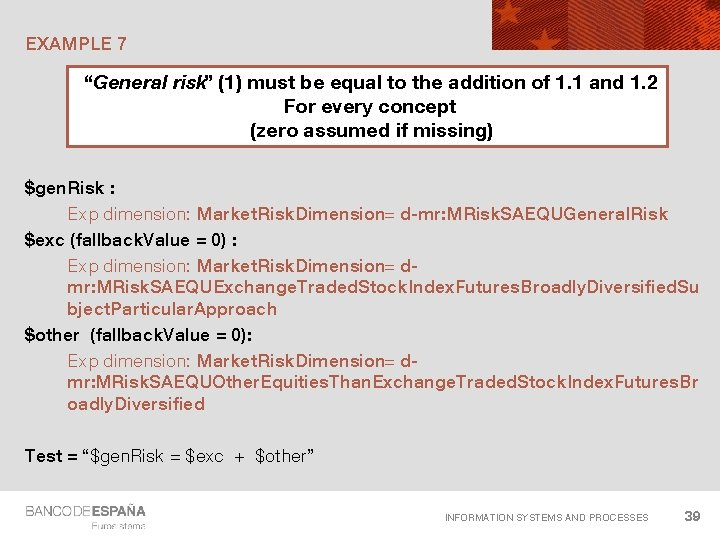EXAMPLE 7 “General risk” (1) must be equal to the addition of 1. 1 and 1. 2 For every concept (zero assumed if missing) \$gen. Risk : Exp dimension: Market. Risk. Dimension= d-mr: MRisk. SAEQUGeneral. Risk \$exc (fallback. Value = 0) : Exp dimension: Market. Risk. Dimension= dmr: MRisk. SAEQUExchange. Traded. Stock. Index. Futures. Broadly. Diversified. Su bject. Particular. Approach \$other (fallback. Value = 0): Exp dimension: Market. Risk. Dimension= dmr: MRisk. SAEQUOther. Equities. Than. Exchange. Traded. Stock. Index. Futures. Br oadly. Diversified Test = “\$gen. Risk = \$exc + \$other” INFORMATION SYSTEMS AND PROCESSES 39EXAMPLE 8 Total “Equities in trading book” equal to the sum of its breakdown For every concept (zero assumed if missing) \$total : Exp dimension: Market. Risk. Dimension= MRisk. SAEQUTotal \$breakdown* (fallback. Value = 0): Exp dimension: Market. Risk. Dimension= d-mr: MRisk. SAEQUGeneral. Risk … d-mr: MRisk. SAEQUOther. Non. Delta. Risks. Options Test = “\$total = sum(\$breakdown)” INFORMATION SYSTEMS AND PROCESSES 40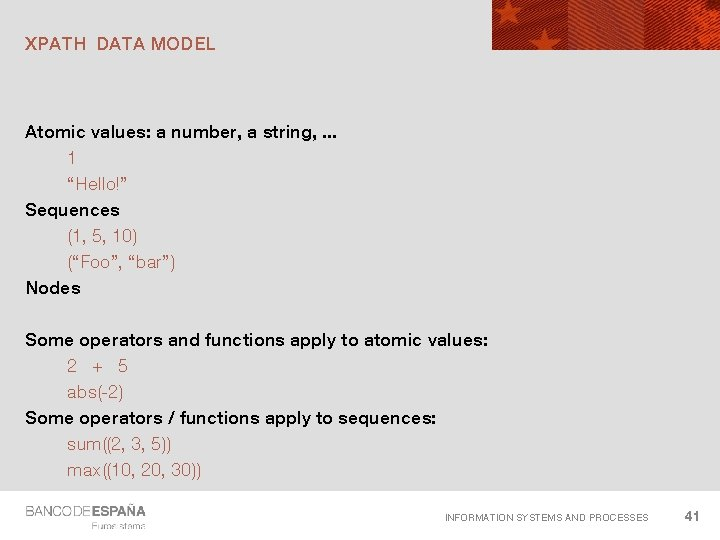XPATH DATA MODEL Atomic values: a number, a string, . . . 1 “Hello!” Sequences (1, 5, 10) (“Foo”, “bar”) Nodes Some operators and functions apply to atomic values: 2 + 5 abs(-2) Some operators / functions apply to sequences: sum((2, 3, 5)) max((10, 20, 30)) INFORMATION SYSTEMS AND PROCESSES 41BIND AS SEQUENCE ATTRIBUTE The attribute “bind. As. Sequence” in a variable is used to make the difference between: - Variables to be bound to single values on each evaluation - Variables to be bound to sequences of values Use sequence functions/operators with sequence variables Use atomic/node functions/ operators with simple variables Sequence variables are to be used with filters that select more than one value. Only aspects explicitly filtered* for these variables are mixed in one evaluation *More precisely: only aspects covered INFORMATION SYSTEMS AND PROCESSES 42EXAMPLE 8 B Total “Equities in trading book” equal to the sum of its breakdown For every concept (zero assumed if missing) \$total : Exp dimension: Market. Risk. Dimension= MRisk. SAEQUTotal \$breakdown* (fallback. Value = 0): Exp dimension: Market. Risk. Dimension= child of MRisk. SAEQUTotal Test = “\$total = sum(\$breakdown)” INFORMATION SYSTEMS AND PROCESSES 43AXIS IN EXPLICIT DIMENSION FILTERS World AXIS POSSIBLE VALUES: child: France, Spain, Belgium Asia Europe descentant: . . . France, Paris, Marseille, Lyon Spain, . . . France Spain Belgium, . . . child-or-self: Europe, France, Spain, Belgium descendant-or-self: Europe Paris Marseille Lyon The tree is selected choosing one extended link role and one arc role France, Paris, Marseille, Lyon Spain, . . . Belgium, . . . COREP and FINREP have hierarchies for every dimension in the default extended link role and domain-member arc role INFORMATION SYSTEMS AND PROCESSES 44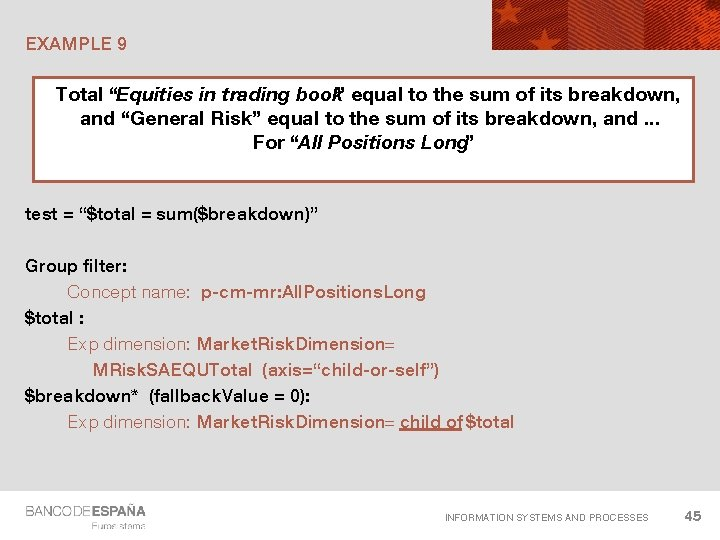EXAMPLE 9 Total “Equities in trading book” equal to the sum of its breakdown, and “General Risk” equal to the sum of its breakdown, and. . . For “All Positions Long” test = “\$total = sum(\$breakdown)” Group filter: Concept name: p-cm-mr: All. Positions. Long \$total : Exp dimension: Market. Risk. Dimension= MRisk. SAEQUTotal (axis=“child-or-self”) \$breakdown* (fallback. Value = 0): Exp dimension: Market. Risk. Dimension= child of \$total INFORMATION SYSTEMS AND PROCESSES 45DIMENSION FILTERS Dimension: Using its name (@qname) Using an XPath expression that returns a name (@qname. Expression) A list of members: Using its name (@qname) Using an XPath expression that returns a name (@qname. Expression) Using a reference to another variable (@variable) + (optional) an axis: Axis value Extended link role Arc role INFORMATION SYSTEMS AND PROCESSES 46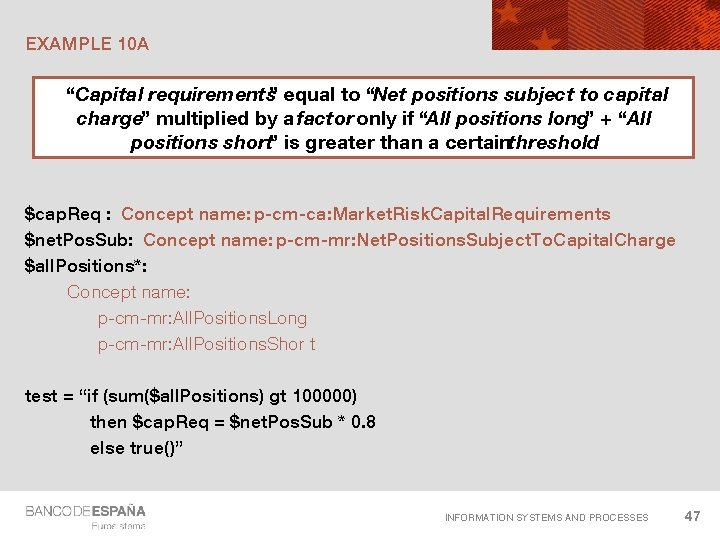EXAMPLE 10 A “Capital requirements” equal to “Net positions subject to capital charge” multiplied by a factor only if “All positions long” + “All positions short” is greater than a certainthreshold \$cap. Req : Concept name: p-cm-ca: Market. Risk. Capital. Requirements \$net. Pos. Sub: Concept name: p-cm-mr: Net. Positions. Subject. To. Capital. Charge \$all. Positions*: Concept name: p-cm-mr: All. Positions. Long p-cm-mr: All. Positions. Shor t test = “if (sum(\$all. Positions) gt 100000) then \$cap. Req = \$net. Pos. Sub * 0. 8 else true()” INFORMATION SYSTEMS AND PROCESSES 47EXAMPLE 10 B “Capital requirements” equal to “Net positions subject to capital charge” multiplied by a factor only if “All positions long” + “All positions short” is greater than a certainthreshold test = “\$cap. Req = \$net. Pos. Sub * 0. 8” \$cap. Req : Concept name: p-cm-ca: Market. Risk. Capital. Requirements \$net. Pos. Sub: Concept name: p-cm-mr: Net. Positions. Subject. To. Capital. Charge \$all. Positions*: Concept name: p-cm-mr: All. Positions. Long p-cm-mr: All. Positions. Shor t PRECONDITION: test = “sum(\$all. Positions) gt 1000000” INFORMATION SYSTEMS AND PROCESSES 48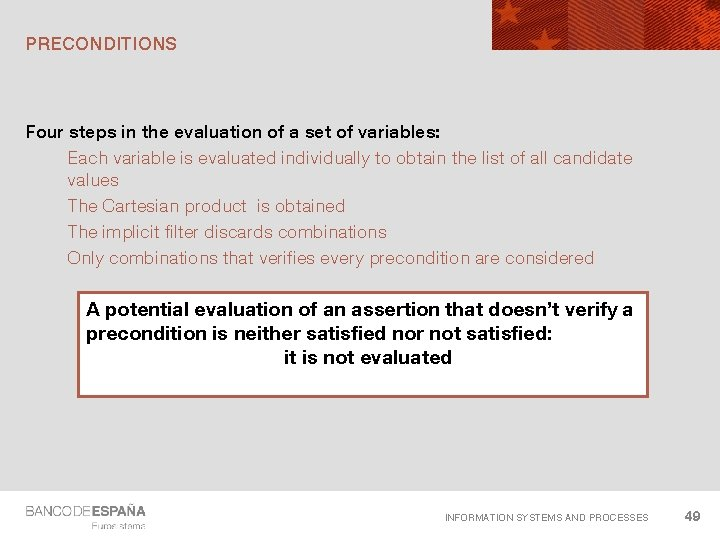PRECONDITIONS Four steps in the evaluation of a set of variables: Each variable is evaluated individually to obtain the list of all candidate values The Cartesian product is obtained The implicit filter discards combinations Only combinations that verifies every precondition are considered A potential evaluation of an assertion that doesn’t verify a precondition is neither satisfied nor not satisfied: it is not evaluated INFORMATION SYSTEMS AND PROCESSES 49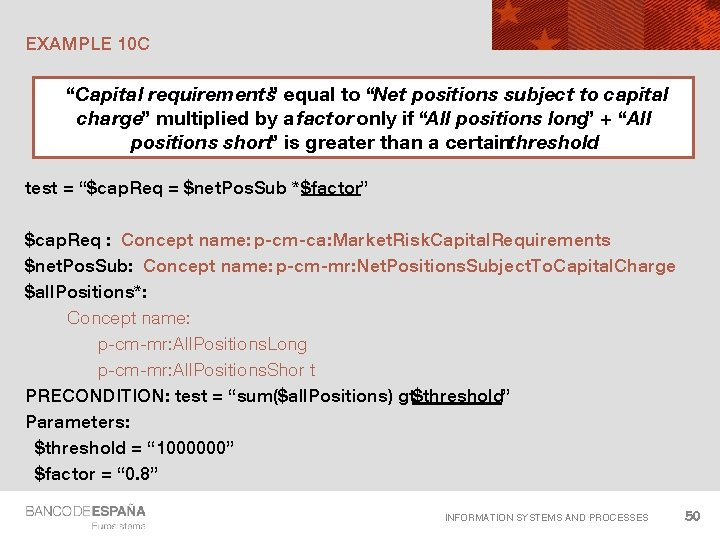EXAMPLE 10 C “Capital requirements” equal to “Net positions subject to capital charge” multiplied by a factor only if “All positions long” + “All positions short” is greater than a certainthreshold test = “\$cap. Req = \$net. Pos. Sub * \$factor” \$cap. Req : Concept name: p-cm-ca: Market. Risk. Capital. Requirements \$net. Pos. Sub: Concept name: p-cm-mr: Net. Positions. Subject. To. Capital. Charge \$all. Positions*: Concept name: p-cm-mr: All. Positions. Long p-cm-mr: All. Positions. Shor t PRECONDITION: test = “sum(\$all. Positions) gt\$threshold” Parameters: \$threshold = “ 1000000” \$factor = “ 0. 8” INFORMATION SYSTEMS AND PROCESSES 50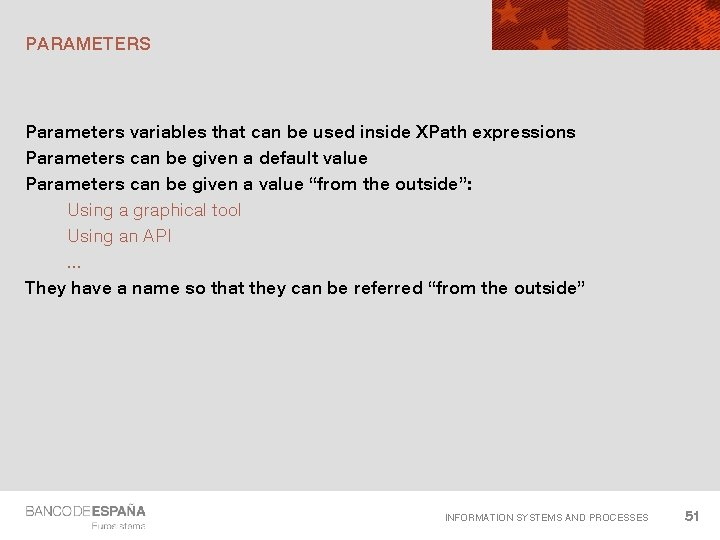PARAMETERS Parameters variables that can be used inside XPath expressions Parameters can be given a default value Parameters can be given a value “from the outside”: Using a graphical tool Using an API. . . They have a name so that they can be referred “from the outside” INFORMATION SYSTEMS AND PROCESSES 51EXAMPLE 11 A: BELGIUM ROUNDING APPROACH Total “Equities in trading book” equal to the sum of its breakdown, and “General Risk” equal to the sum of its breakdown, and. . . For “All Positions Long” considering rounding error test = “abs(\$total - sum(\$breakdown) ) le \$threshold” Group filter: Concept name: p-cm-mr: All. Positions. Long \$total : Exp dimension: Market. Risk. Dimension= MRisk. SAEQUTotal (axis=“child-or-self”) \$breakdown* (fallback. Value = 0): Exp dimension: Market. Risk. Dimension= child of \$total Parameter \$threshold = 1 INFORMATION SYSTEMS AND PROCESSES 52EXAMPLE 11 B: SPANISH ROUNDING APPROACH Total “Equities in trading book” equal to the sum of its breakdown, and “General Risk” equal to the sum of its breakdown, and. . . For “All Positions Long” considering rounding error test = “abs(\$total - sum(\$breakdown) ) le \$threshold” Group filter: Concept name: p-cm-mr: All. Positions. Long \$total : Exp dimension: Market. Risk. Dimension= MRisk. SAEQUTotal (axis=“child-or-self”) \$breakdown* (fallback. Value = 0): Exp dimension: Market. Risk. Dimension= child of \$total General variable: \$threshold = “ 1000 * (count(\$breakdown) + 1) div 2” INFORMATION SYSTEMS AND PROCESSES 53GENERAL VARIABLES Its value is the result of evaluating an XPath expression They can make reference to other variables/parameters in the assertion / formula Can be used for intermediate values that are used repeatedly in the assertion / formla INFORMATION SYSTEMS AND PROCESSES 54VARIABLES/PARAMETERS COMPARISON TABLE Fact Variables Parameters General variables Aim Bind facts in instance Parameterize the behaviour of some formula/assertions Intermediate results derived from other variables Iterations in complex formulae Use filters Yes No No Implicit filter applies Yes No No Can be given values from outside the processor No Yes May (using external functions) Can have a fallback value Yes No No Can bind as sequence / Yes single No Yes Fixed value throughout the processing No Yes No References to other variables Yes (through filters) No Yes INFORMATION SYSTEMS AND PROCESSES 55TECHNICAL REPRESENTATION Value. Assertion @complement = “false” @test = ““\$a ge \$b * \$c” @aspect. Model = “dimensional” @implicit. Filtering = “true” 0. . n Group filter @name=“a” 0. . n Parameter @bind. As. Sequence=“false” @name = “My. Threshold” 0. . n Fact Variable @fallback. Value=“ 0” @bind. As. Sequence=“false” 0. . n General Variable @select=“\$a * \$b” @bind. As. Sequence=“false” @complement = “false” @cover = “true” Precondition @test=“. . . ” @name=“c” @name=“b” 0. . n Variable filter INFORMATION SYSTEMS AND PROCESSES 56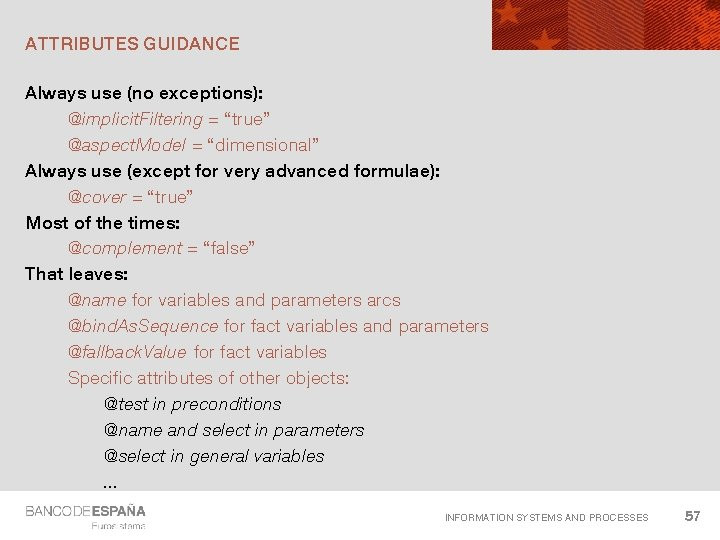ATTRIBUTES GUIDANCE Always use (no exceptions): @implicit. Filtering = “true” @aspect. Model = “dimensional” Always use (except for very advanced formulae): @cover = “true” Most of the times: @complement = “false” That leaves: @name for variables and parameters arcs @bind. As. Sequence for fact variables and parameters @fallback. Value for fact variables Specific attributes of other objects: @test in preconditions @name and select in parameters @select in general variables. . . INFORMATION SYSTEMS AND PROCESSES 57EXAMPLE 12 Check that “Capital Requirements” for “Other non-delta risks for options” is not reported test = “false()” \$cap. Req : Concept name: p-cm-ca: Market. Risk. Capital. Requirements Exp dimension: Market. Risk. Dimension = MRisk. SAEQUOther. Non. Delta. Risks. Options INFORMATION SYSTEMS AND PROCESSES 58EXAMPLE 13 Check that “Capital Requirements” for “Other non-delta risks for options” must be reported test = “true()” \$cap. Req : Concept name: p-cm-ca: Market. Risk. Capital. Requirements Exp dimension: Market. Risk. Dimension = MRisk. SAEQUOther. Non. Delta. Risks. Options INFORMATION SYSTEMS AND PROCESSES 59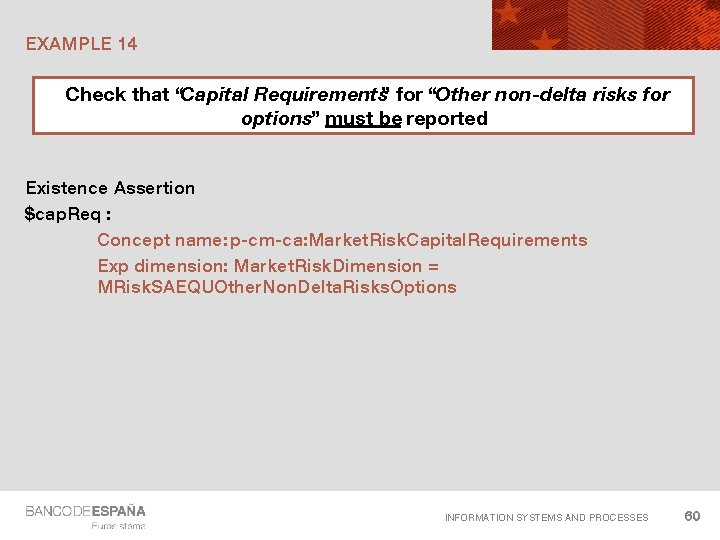EXAMPLE 14 Check that “Capital Requirements” for “Other non-delta risks for options” must be reported Existence Assertion \$cap. Req : Concept name: p-cm-ca: Market. Risk. Capital. Requirements Exp dimension: Market. Risk. Dimension = MRisk. SAEQUOther. Non. Delta. Risks. Options INFORMATION SYSTEMS AND PROCESSES 60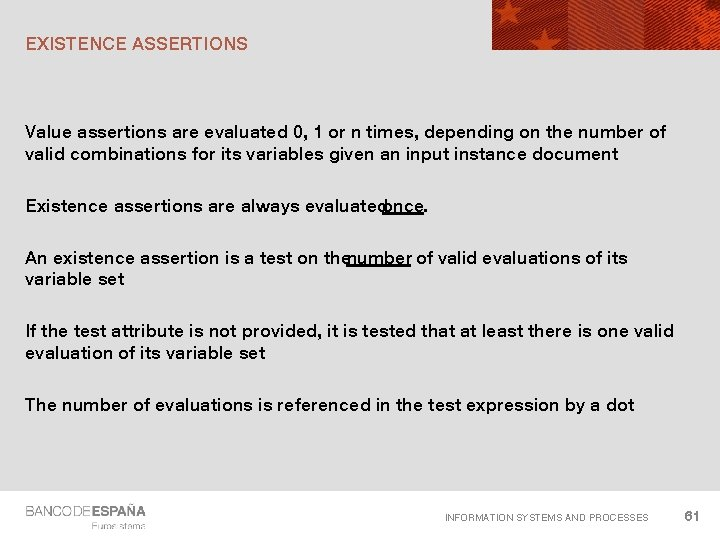EXISTENCE ASSERTIONS Value assertions are evaluated 0, 1 or n times, depending on the number of valid combinations for its variables given an input instance document Existence assertions are always evaluatedonce. An existence assertion is a test on thenumber of valid evaluations of its variable set If the test attribute is not provided, it is tested that at least there is one valid evaluation of its variable set The number of evaluations is referenced in the test expression by a dot INFORMATION SYSTEMS AND PROCESSES 61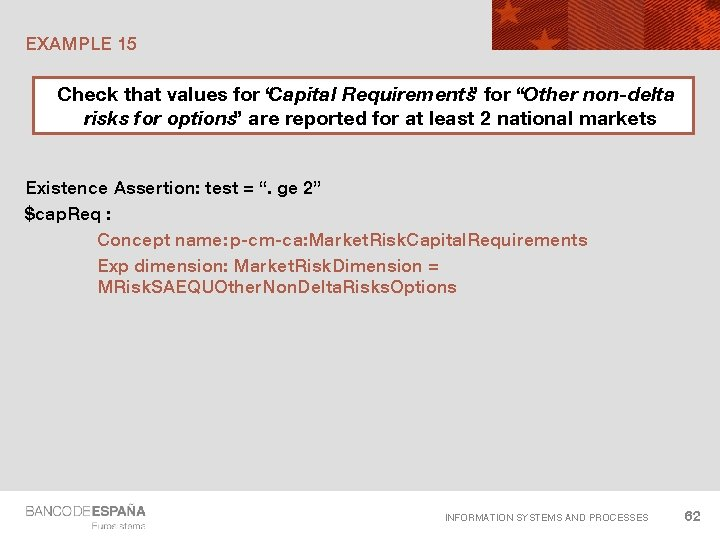EXAMPLE 15 Check that values for “Capital Requirements” for “Other non-delta risks for options” are reported for at least 2 national markets Existence Assertion: test = “. ge 2” \$cap. Req : Concept name: p-cm-ca: Market. Risk. Capital. Requirements Exp dimension: Market. Risk. Dimension = MRisk. SAEQUOther. Non. Delta. Risks. Options INFORMATION SYSTEMS AND PROCESSES 62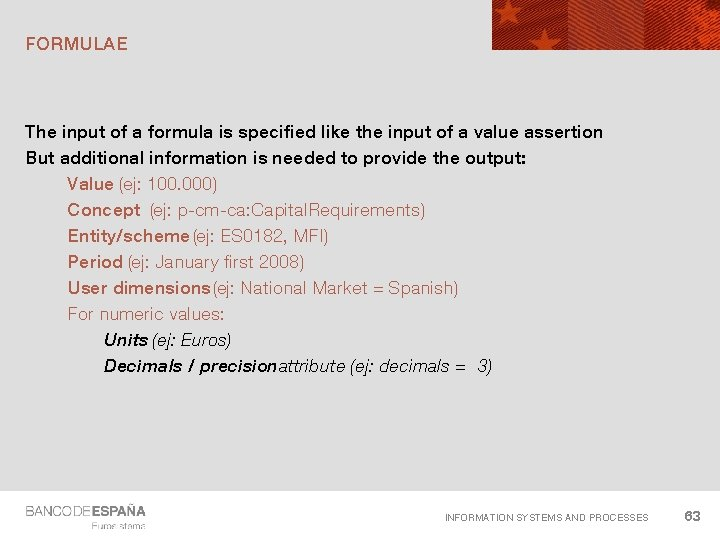FORMULAE The input of a formula is specified like the input of a value assertion But additional information is needed to provide the output: Value (ej: 100. 000) Concept (ej: p-cm-ca: Capital. Requirements) Entity/scheme (ej: ES 0182, MFI) Period (ej: January first 2008) User dimensions (ej: National Market = Spanish) For numeric values: Units (ej: Euros) Decimals / precisionattribute (ej: decimals = -3) INFORMATION SYSTEMS AND PROCESSES 63FORMULAE Value must always be explicitly defined Decimals/precision have a default value (precision 0) Aspects (concept, entity, period, user dimensions, units, . . . ) can be: Explicitly defined Defined in terms of input facts (source) INFORMATION SYSTEMS AND PROCESSES 64EXAMPLE 16: SIMPLE FORMULA “Capital requirements” can be calculated as “Net positions subject to capital charge ” multiplied by 0. 8 Input : \$net. Pos. Sub. Cap. Ch: Concept name: p-cm-mr: Net. Positions. Subject. To. Capital. Charge \$decimals: Parameter Aspects not explicitly expressed in the output are “copied” from the source: - Unit - Period - Entity. . . Output: value = “\$net. Pos. Sub. Cap. Ch * 0. 8” decimals = “\$decimals” Source = “net. Pos. Sub. Cap. Ch” Concept name: p-cm-ca: Market. Risk. Capital. Requirements INFORMATION SYSTEMS AND PROCESSES 65EXAMPLE 17: SIMPLE FORMULA WITH FALLBACK “Net positions subject to capital charge ” calculated as the addition of short and long net positions (zero assumed if not reported) Input : \$short. Net (fallback value = 0): Concept name: p-cm-mr: Net. Positions. Long \$long. Net (fallback value = 0): Concept name: p-cm-mr: Net. Positions. Short If the source variable falls back, aspects Output: cannot be obtained. value = “\$short. Net + \$long. Net” The fallback value is a number !!! The processor will raise an error decimals = “\$decimals” Source = “\$short. Net” Concept name p-cm-mr: Net. Positions. Subject. To. Capital. Charge INFORMATION SYSTEMS AND PROCESSES 66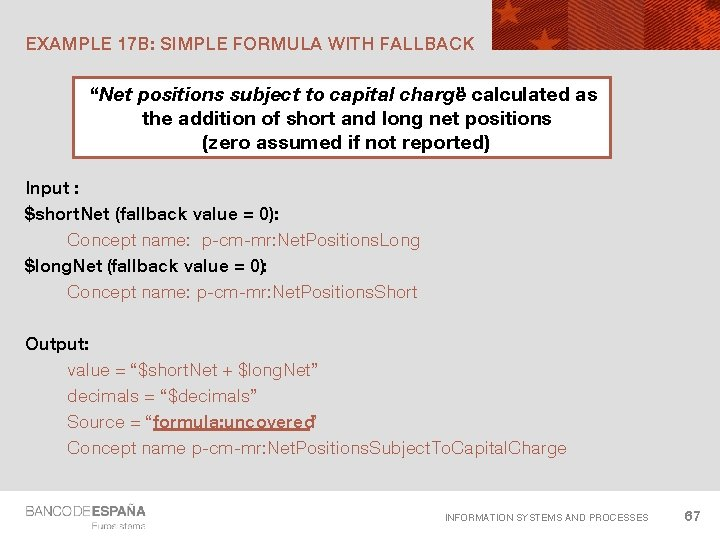EXAMPLE 17 B: SIMPLE FORMULA WITH FALLBACK “Net positions subject to capital charge ” calculated as the addition of short and long net positions (zero assumed if not reported) Input : \$short. Net (fallback value = 0): Concept name: p-cm-mr: Net. Positions. Long \$long. Net (fallback value = 0): Concept name: p-cm-mr: Net. Positions. Short Output: value = “\$short. Net + \$long. Net” decimals = “\$decimals” Source = “formula: uncovered” Concept name p-cm-mr: Net. Positions. Subject. To. Capital. Charge INFORMATION SYSTEMS AND PROCESSES 67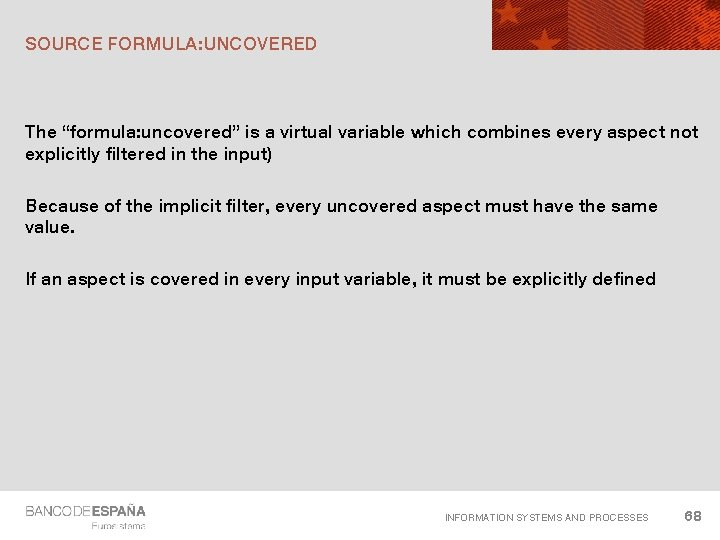SOURCE FORMULA: UNCOVERED The “formula: uncovered” is a virtual variable which combines every aspect not explicitly filtered in the input) Because of the implicit filter, every uncovered aspect must have the same value. If an aspect is covered in every input variable, it must be explicitly defined INFORMATION SYSTEMS AND PROCESSES 68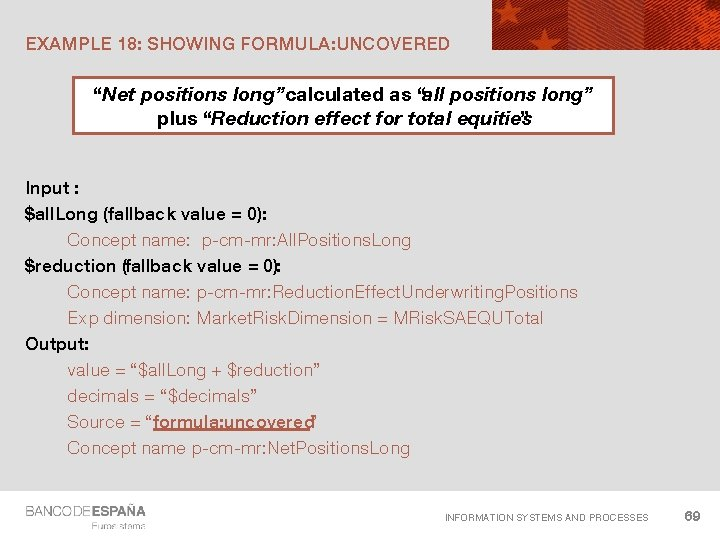EXAMPLE 18: SHOWING FORMULA: UNCOVERED “Net positions long”calculated as “all positions long” plus “Reduction effect for total equities ” Input : \$all. Long (fallback value = 0): Concept name: p-cm-mr: All. Positions. Long \$reduction (fallback value = 0): Concept name: p-cm-mr: Reduction. Effect. Underwriting. Positions Exp dimension: Market. Risk. Dimension = MRisk. SAEQUTotal Output: value = “\$all. Long + \$reduction” decimals = “\$decimals” Source = “formula: uncovered” Concept name p-cm-mr: Net. Positions. Long INFORMATION SYSTEMS AND PROCESSES 69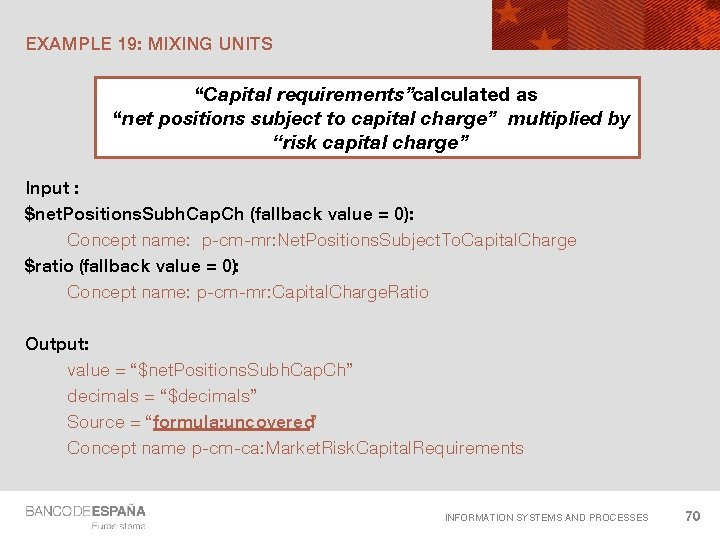EXAMPLE 19: MIXING UNITS “Capital requirements”calculated as “net positions subject to capital charge” multiplied by “risk capital charge” Input : \$net. Positions. Subh. Cap. Ch (fallback value = 0): Concept name: p-cm-mr: Net. Positions. Subject. To. Capital. Charge \$ratio (fallback value = 0): Concept name: p-cm-mr: Capital. Charge. Ratio Output: value = “\$net. Positions. Subh. Cap. Ch” decimals = “\$decimals” Source = “formula: uncovered” Concept name p-cm-ca: Market. Risk. Capital. Requirements INFORMATION SYSTEMS AND PROCESSES 70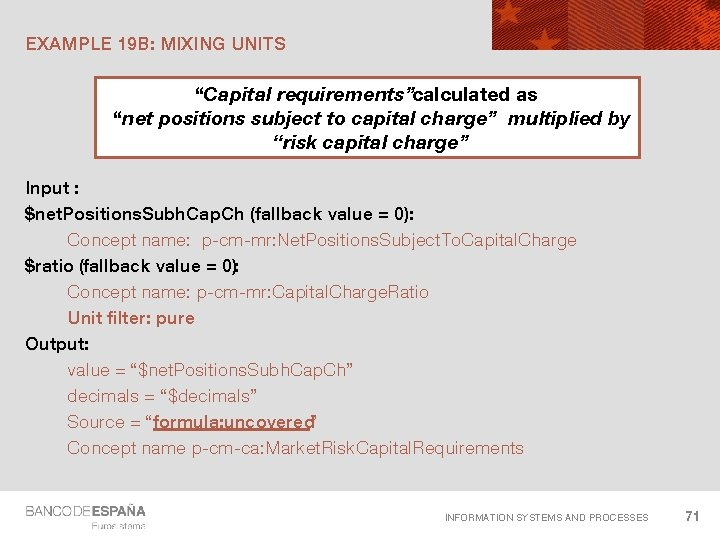EXAMPLE 19 B: MIXING UNITS “Capital requirements”calculated as “net positions subject to capital charge” multiplied by “risk capital charge” Input : \$net. Positions. Subh. Cap. Ch (fallback value = 0): Concept name: p-cm-mr: Net. Positions. Subject. To. Capital. Charge \$ratio (fallback value = 0): Concept name: p-cm-mr: Capital. Charge. Ratio Unit filter: pure Output: value = “\$net. Positions. Subh. Cap. Ch” decimals = “\$decimals” Source = “formula: uncovered” Concept name p-cm-ca: Market. Risk. Capital. Requirements INFORMATION SYSTEMS AND PROCESSES 71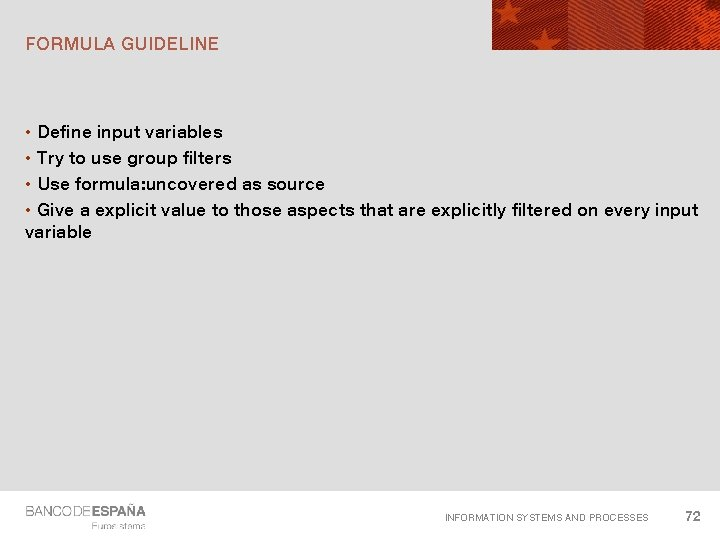FORMULA GUIDELINE • Define input variables • Try to use group filters • Use formula: uncovered as source • Give a explicit value to those aspects that are explicitly filtered on every input variable INFORMATION SYSTEMS AND PROCESSES 72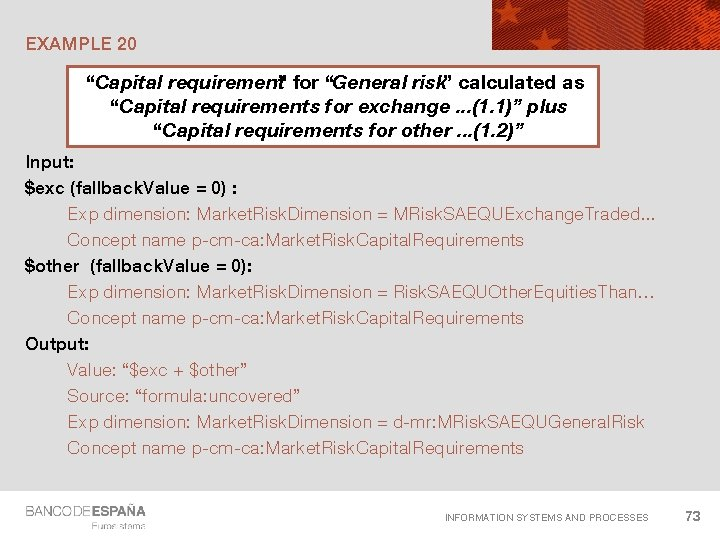EXAMPLE 20 “Capital requirement” for “General risk” calculated as “Capital requirements for exchange. . . (1. 1)” plus “Capital requirements for other. . . (1. 2)” Input: \$exc (fallback. Value = 0) : Exp dimension: Market. Risk. Dimension = MRisk. SAEQUExchange. Traded. . . Concept name p-cm-ca: Market. Risk. Capital. Requirements \$other (fallback. Value = 0): Exp dimension: Market. Risk. Dimension = Risk. SAEQUOther. Equities. Than… Concept name p-cm-ca: Market. Risk. Capital. Requirements Output: Value: “\$exc + \$other” Source: “formula: uncovered” Exp dimension: Market. Risk. Dimension = d-mr: MRisk. SAEQUGeneral. Risk Concept name p-cm-ca: Market. Risk. Capital. Requirements INFORMATION SYSTEMS AND PROCESSES 73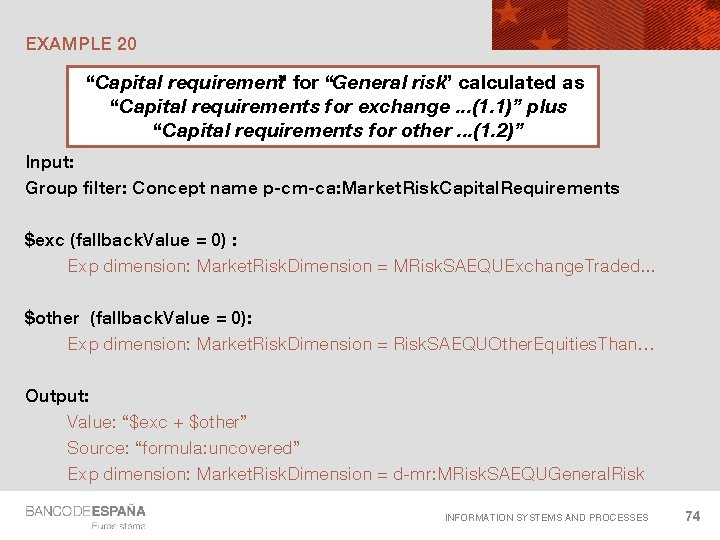EXAMPLE 20 “Capital requirement” for “General risk” calculated as “Capital requirements for exchange. . . (1. 1)” plus “Capital requirements for other. . . (1. 2)” Input: Group filter: Concept name p-cm-ca: Market. Risk. Capital. Requirements \$exc (fallback. Value = 0) : Exp dimension: Market. Risk. Dimension = MRisk. SAEQUExchange. Traded. . . \$other (fallback. Value = 0): Exp dimension: Market. Risk. Dimension = Risk. SAEQUOther. Equities. Than… Output: Value: “\$exc + \$other” Source: “formula: uncovered” Exp dimension: Market. Risk. Dimension = d-mr: MRisk. SAEQUGeneral. Risk INFORMATION SYSTEMS AND PROCESSES 74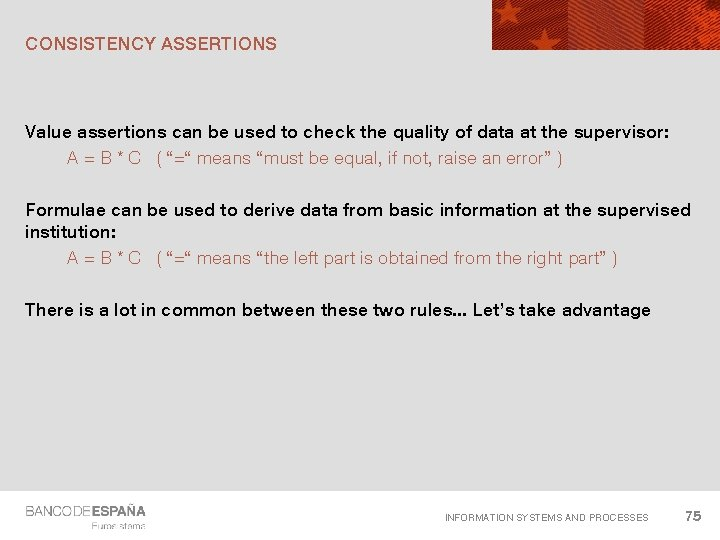CONSISTENCY ASSERTIONS Value assertions can be used to check the quality of data at the supervisor: A = B * C ( “=“ means “must be equal, if not, raise an error” ) Formulae can be used to derive data from basic information at the supervised institution: A = B * C ( “=“ means “the left part is obtained from the right part” ) There is a lot in common between these two rules. . . Let’s take advantage INFORMATION SYSTEMS AND PROCESSES 75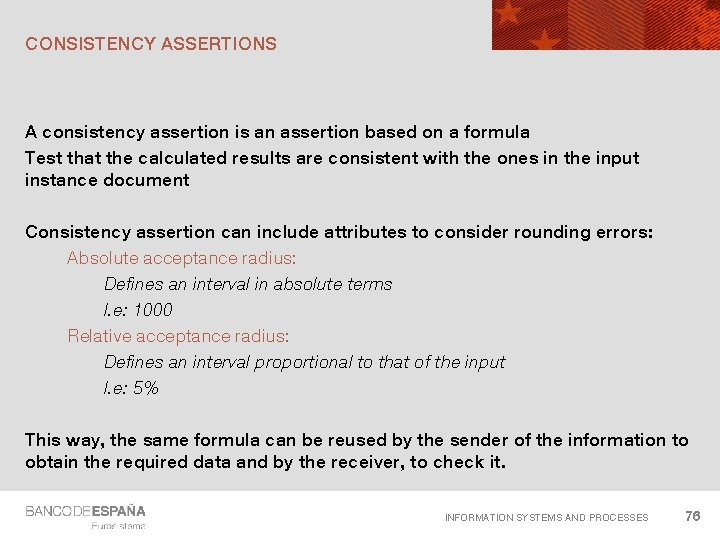CONSISTENCY ASSERTIONS A consistency assertion is an assertion based on a formula Test that the calculated results are consistent with the ones in the input instance document Consistency assertion can include attributes to consider rounding errors: Absolute acceptance radius: Defines an interval in absolute terms I. e: 1000 Relative acceptance radius: Defines an interval proportional to that of the input I. e: 5% This way, the same formula can be reused by the sender of the information to obtain the required data and by the receiver, to check it. INFORMATION SYSTEMS AND PROCESSES 76A LOT OF FILTERS AVAILABLE Concept aspect: By name By period-type By balance attribute By custom-attribute By data-type. . . Dimensions: Explicit dimensions Typed dimensions General filters: Value Precision. . Unit: Single measure General measure Period: General Period-start Period-end Period-instant Forever filter Instant-duration Tuple Entity Segment / scenario. . . INFORMATION SYSTEMS AND PROCESSES 77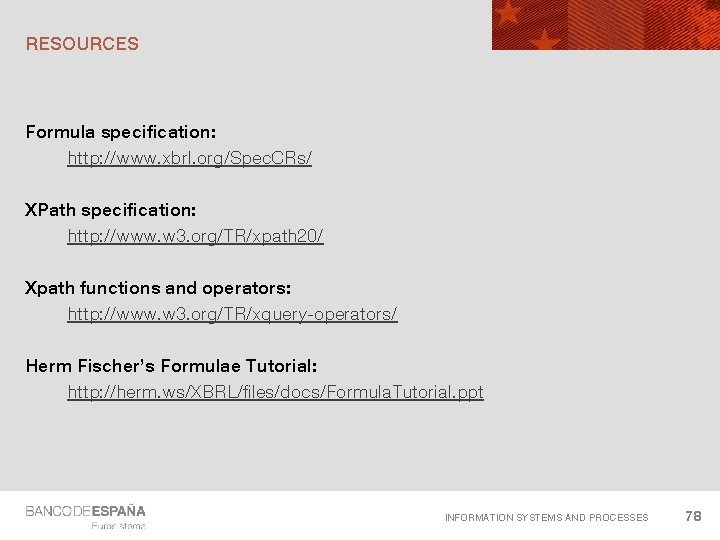RESOURCES Formula specification: http: //www. xbrl. org/Spec. CRs/ XPath specification: http: //www. w 3. org/TR/xpath 20/ Xpath functions and operators: http: //www. w 3. org/TR/xquery-operators/ Herm Fischer’s Formulae Tutorial: http: //herm. ws/XBRL/files/docs/Formula. Tutorial. ppt INFORMATION SYSTEMS AND PROCESSES 78BANK OF SPAIN PROJECT DETAILS INFORMATION SYSTEMS AND PROCESSES 79NEXT STEPS INFORMATION SYSTEMS AND PROCESSES 80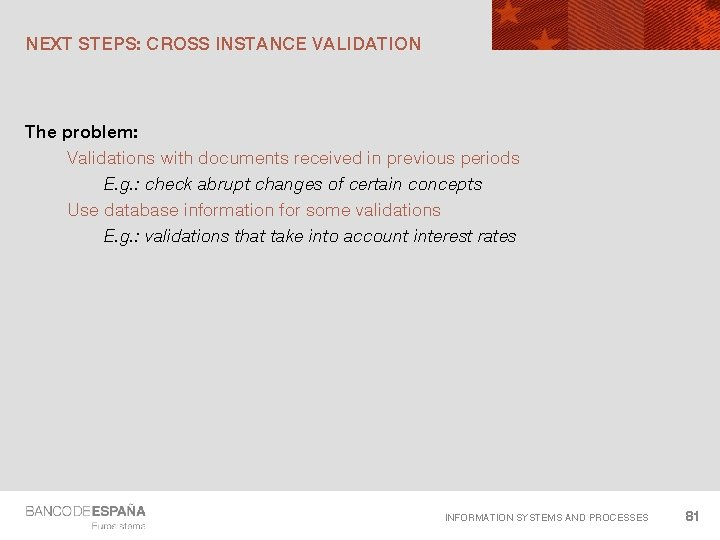NEXT STEPS: CROSS INSTANCE VALIDATION The problem: Validations with documents received in previous periods E. g. : check abrupt changes of certain concepts Use database information for some validations E. g. : validations that take into account interest rates INFORMATION SYSTEMS AND PROCESSES 81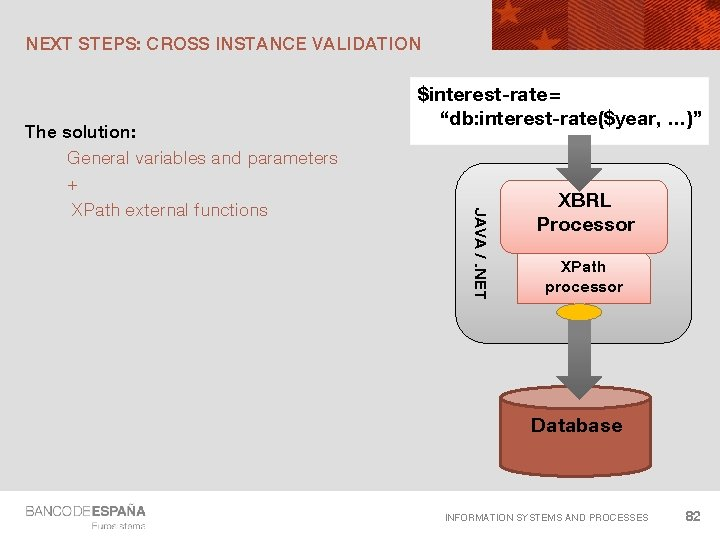NEXT STEPS: CROSS INSTANCE VALIDATION JAVA /. NET The solution: General variables and parameters + XPath external functions \$interest-rate = “db: interest-rate(\$year, …)” XBRL Processor XPath processor Database INFORMATION SYSTEMS AND PROCESSES 82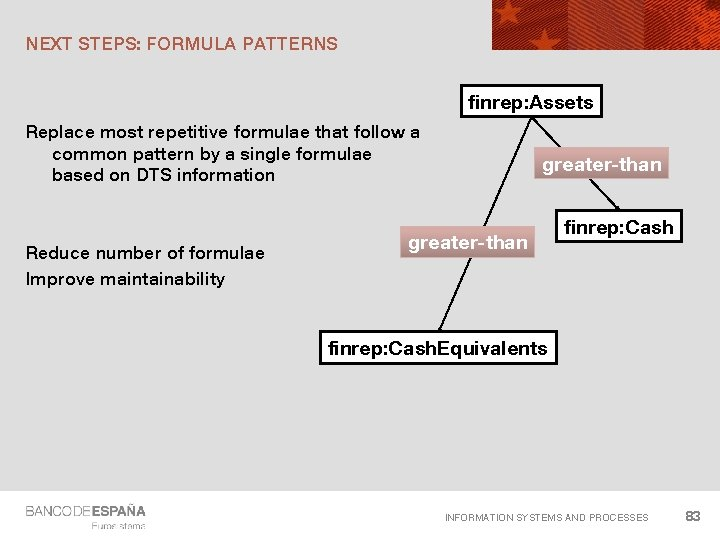NEXT STEPS: FORMULA PATTERNS finrep: Assets Replace most repetitive formulae that follow a common pattern by a single formulae based on DTS information Reduce number of formulae Improve maintainability greater-than finrep: Cash. Equivalents INFORMATION SYSTEMS AND PROCESSES 83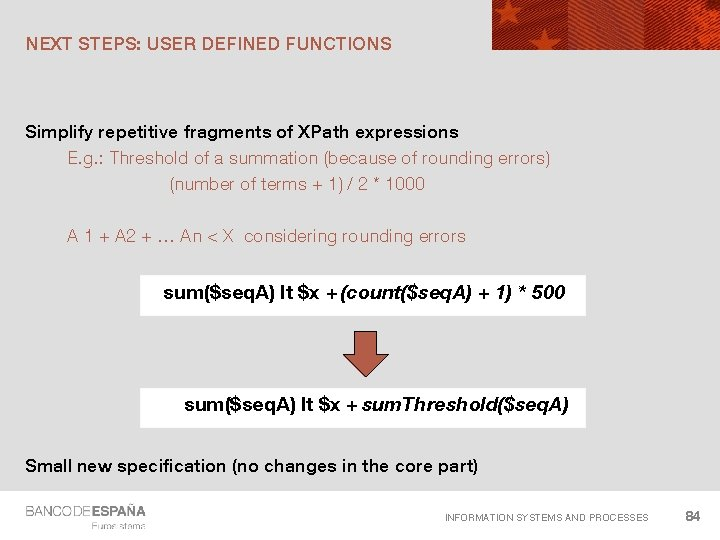NEXT STEPS: USER DEFINED FUNCTIONS Simplify repetitive fragments of XPath expressions E. g. : Threshold of a summation (because of rounding errors) (number of terms + 1) / 2 * 1000 A 1 + A 2 + … An < X considering rounding errors sum(\$seq. A) lt \$x + (count(\$seq. A) + 1) * 500 sum(\$seq. A) lt \$x + sum. Threshold(\$seq. A) Small new specification (no changes in the core part) INFORMATION SYSTEMS AND PROCESSES 84NEXT STEPS: BETTER ERROR REPORTING Current error reporting solution: Generic labels linked to assertions More complex messages: Total assets reported ( 1, 200, 000 € ) are 200% higher than the value reported last year Small new specification (no changes in the core part) INFORMATION SYSTEMS AND PROCESSES 85NEXT CHALLENGES Formula chaining Advanced control of assertions firing Very large instance validation New filters INFORMATION SYSTEMS AND PROCESSES 86BENEFITS Powerful and flexible solution Better maintainability Striking reduction in the number of rules to maintain: ≈ 5300 business rules expressed using ≈ 700 XBRL Formulas Reusability between different actors Regulators and credit institutions (consistency assertions) Different countries: European rules Domestic ones Data available sooner and improved quality Standard and formal language to express formulae Validation at the source of information Market tools available Support from software vendors INFORMATION SYSTEMS AND PROCESSES 87QUESTIONS INFORMATION SYSTEMS AND PROCESSES 88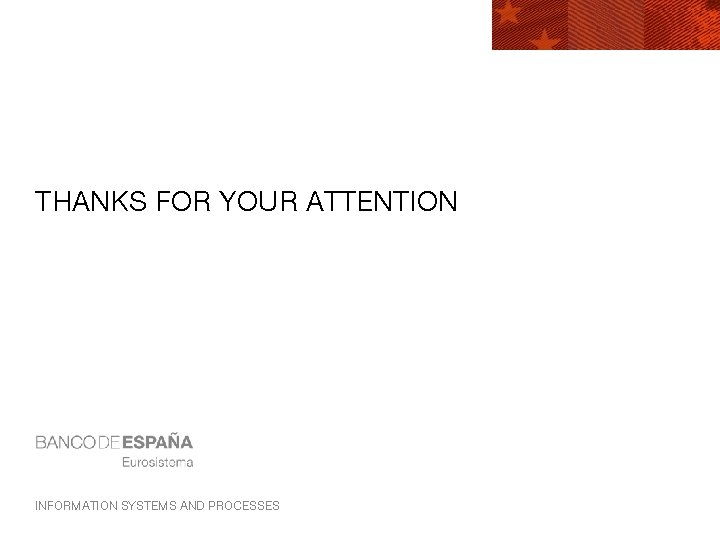THANKS FOR YOUR ATTENTION INFORMATION SYSTEMS AND PROCESSES